# 如何在仿真研究中使用声学拓扑优化

2016年 11月 3日

### 拓扑优化中的目标函数

\min_{\chi} F (\chi)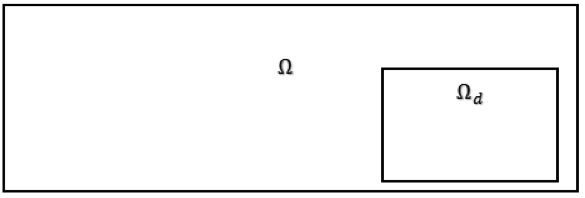### 静态结构力学示例

0 < \chi \leq 1\ \forall\ (x, y)\ \varepsilon\ \Omega_d.

COMSOL Multiphysics 中用于静态结构力学的拓扑优化是 COMSOL 博客先前讨论过的主题。简要概述：研究 MBB 梁，目的是在给定载荷和边界条件下，通过将总应变能降至最低来使刚度最大化。设计域是整个有限元域的一部分。约束应用于结构的总质量。在设计空间中，杨氏模量通过设计变量 \chi 进行插值

E(\chi) = \left\{ \begin{array}{ll}E_0\ \textrm{for}\ \chi=1\\0\ \textrm{for}\ \chi=0 \end{array} \right..

E (\chi) = \chi^p E_0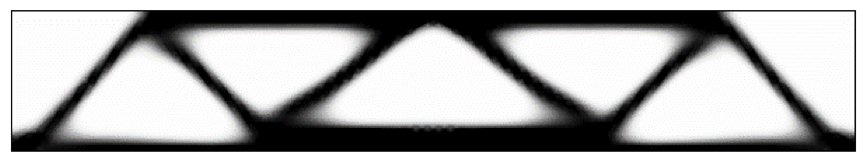### 在 COMSOL Multiphysics® 中执行声学拓扑优化

\rho(\chi) = \left\{ \begin{array}{ll}\rho_2\ \textrm{for}\ \chi=1 \\ \rho_1\ \textrm{for} \ \chi=0 \end{array} \right.

K(\chi) = \left\{ \begin{array}{ll}K_2\ \textrm{for}\ \chi=1\\K_1\ \textrm{for}\ \chi=0 \end{array} \right..

\int^{}_{\Omega_d} \chi d \Omega_d \leq S_r

#### 单目标、单频示例

TL = 10 \log_{10} \left(\frac{W_i}{W_o} \right).

\min_{\chi} TL (420 \text {Hz}).

\int^{}_{\Omega_d} \chi d \Omega_d \leq 0.05.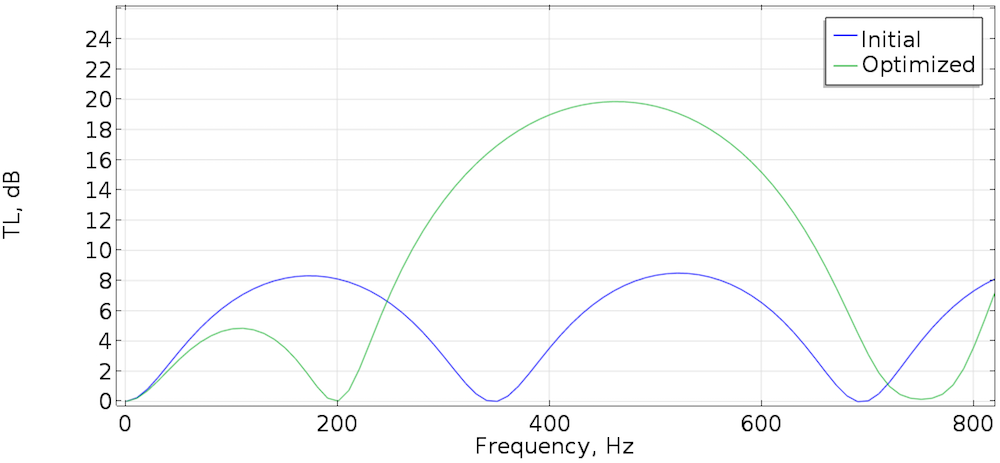#### 双目标、双频示例

1. 将频率 f1/sub> 下的声压级降至最低，并
2. 将频率 f2= 1.5 f1 下的声压级降至最低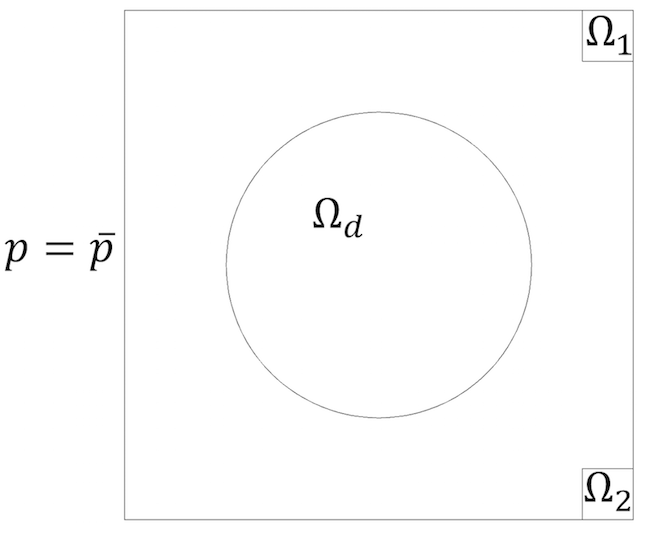\begin{align} \min_{\chi} \max_{f_1 f_2} SPL_i (\chi, f_i) \\ \text {subject to} \int^{}_{\Omega_d} \chi d\Omega_d \leq 0.1. \end{align}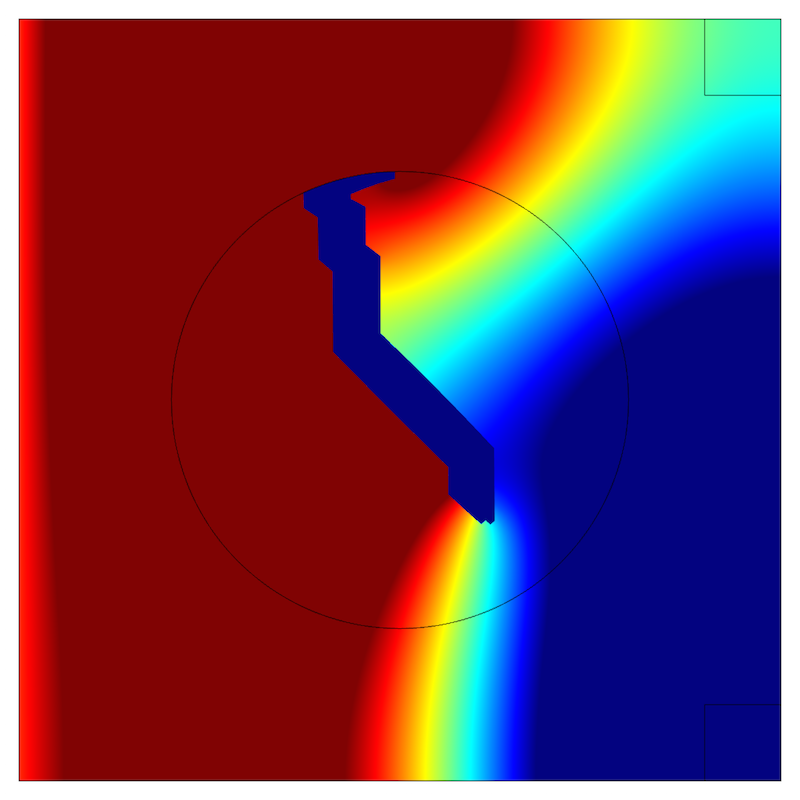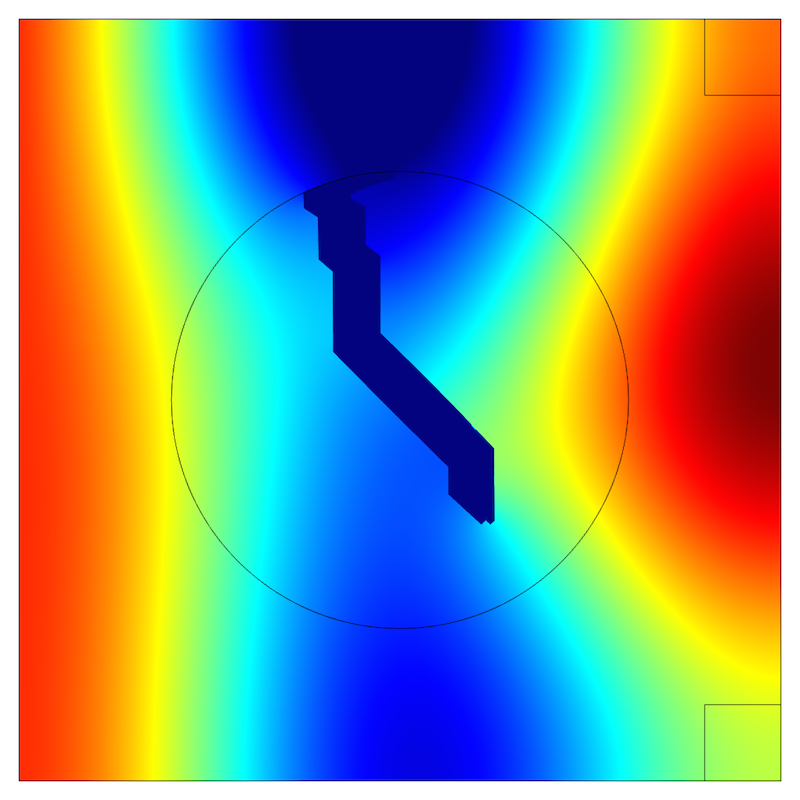#### 单目标、多频示例

\begin{align} \min_{\chi} \sum_{i=1}^{5} (\mid p_i (\chi, f_i, 0, 0.4) \mid -\overline{p}_{obj})^2 \\ \text {subject to} \int^{}_{\Omega_d} \chi d\Omega_d \leq 0.1. \end{align}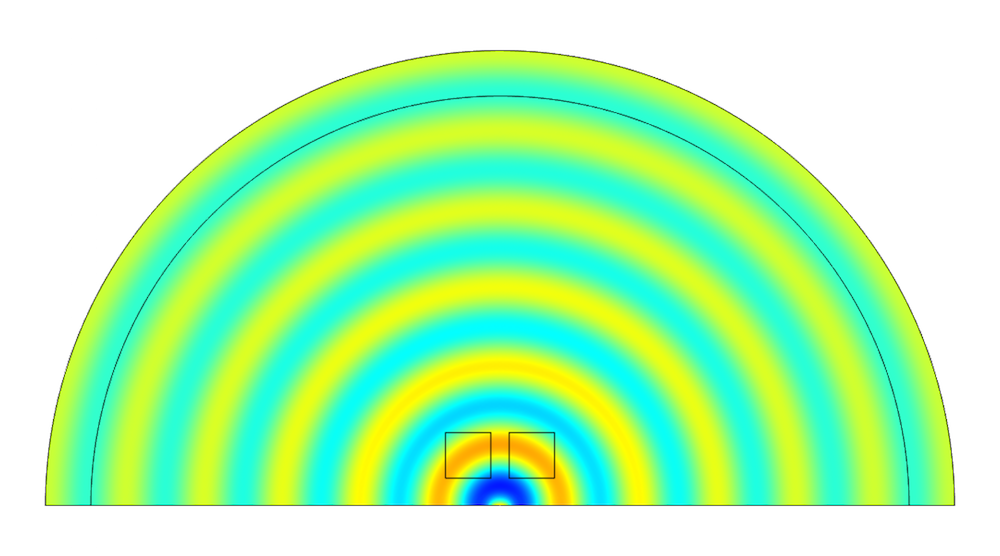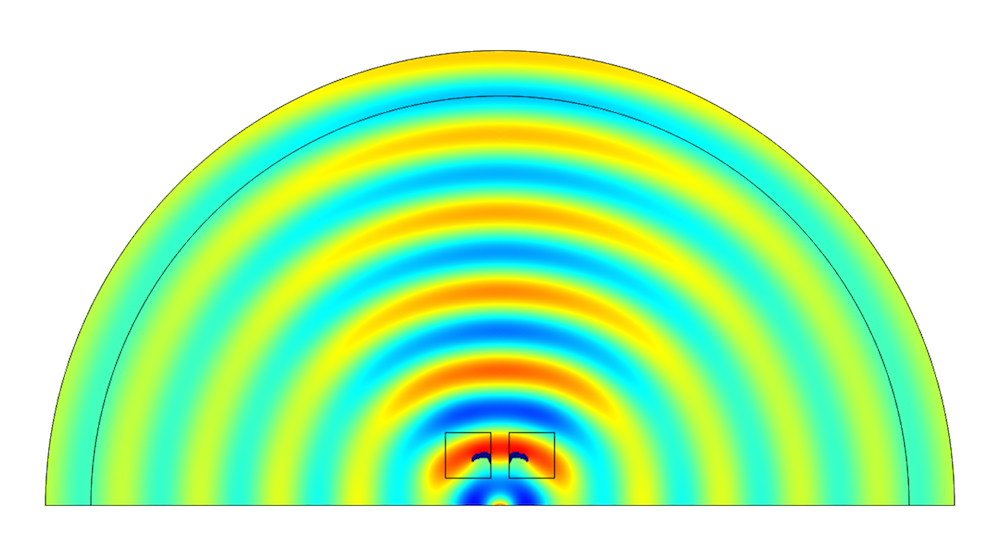4 kHz 下初始状态（左）和优化状态（右）的声压，优化的拓扑在方形设计域中以蓝色显示。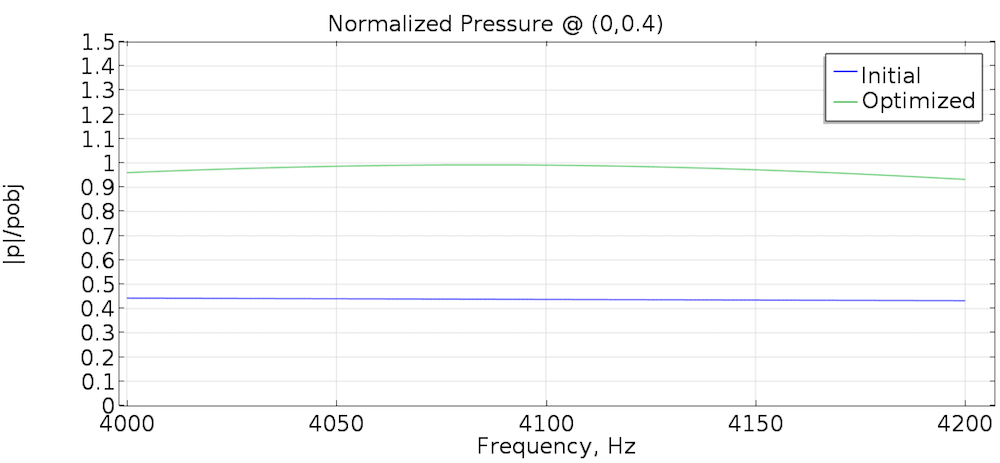### 参考文献

• M.P. Bendsoe, O. Sigmund, Topology Optimization: Theory, Methods, and Applications, Springer 2003.

• T.W. Wu, G.C. Wan, Muffler, “Performance studies and using a direct mixed-body boundary element method and a three-point method for evaluating transmission loss”, Trans. ASME: J. Vib. Acoust. 118 (1996) 479-484.

• Z. Tao, A.F. Seybert, “A review of current techniques for measuring muffler transmission loss”, SAE International, 2003.

### 客座作者简介

René Christensen 在振动声学领域工作了十余年，既担任顾问（iCapture ApS），也是助听器行业的工程技术人员（Otion A/S，瑞声达听力集团）。他对微声学中的热粘性效应建模特别感兴趣，这也是他博士学位的课题。René 于 2015 年加入瑞声达听力集团的硬件平台声学研发团队，担任高级声学工程师，他在这个职位上致力于助听器的设计和优化。

#### 评论 (2)

##### 留言##### ZHOU SHIWEN
2019-06-03

Hello, I am a graduate student. My research direction is about the topology optimization of muffler structure. And I am interested in the simple expansion chamber case in your article. If it’s convenient, could you send me a case file of comsol? My mailbox is 179199061@qq.com.##### ZHOU SHIWEN
2019-06-03

Hello, I am a graduate student. My research direction is about the topology optimization of muffler structure. I am interested in the simple expansion chamber case in your article. If it’s convenient, could you send me a case file of comsol? My mailbox is 1791899061@qq.com. （Sorry for the mistake in the mailbox just now. This is my mailbox. ）